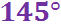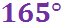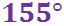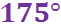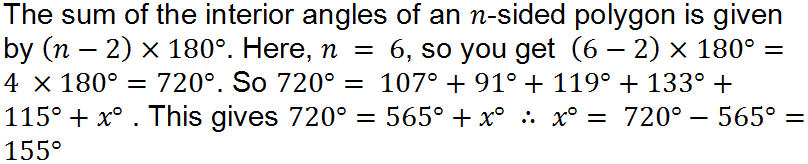Do you recognise these polygons?

# Polygons (F)

A POLYGON is the family name for two-dimensional shapes whose edges are all straight lines. There are a number of properties that apply to all polygons, and a few more that only apply to regular polygons. Test how well you know your facts in this GCSE Maths quiz!

The word polygon derives from the Greek, with ‘poly’ meaning many, and ‘gon’ meaning corner or angle. The prefix ‘poly’ appears in many other words we use, can you think of any? Polystyrene means a chain of many styrene molecules, other chemical names like polyester and polythene have the same logic.

Individual polygons are named according to the number of sides they have, and you should know the names of them up to at least 10 sides.

They mostly have a Greek prefix, such as ‘penta’ for 5, ‘hex’ for 6 and so on. Many of these prefixes appear in other words, which should help you remember them. Think of octopus, heptathlete, decathlon, pentagram… Once there are lots of sides it is easier to write using the digits, such as 20-gon.

All polygons can be divided up into triangles. The easiest way to do this is to start at a corner, and draw diagonals to all the other corners. How many triangles are formed? It will always be 2 less than the number of sides. This is useful as it allows us to calculate the sum of the interior angles, all we have to remember is that there are 180o in a triangle!

At every corner, the sum of the interior and exterior angles will always be 180o. This leads to the result that the sum of the exterior angles in a polygon is 360o.

There are a few more properties that apply tor regular polygons, can you remember what they are?

Question 1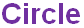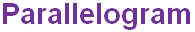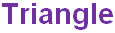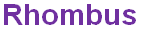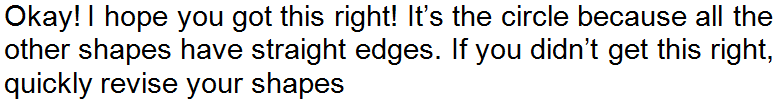Question 2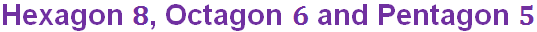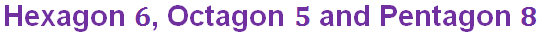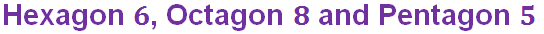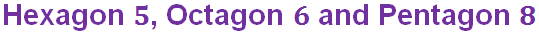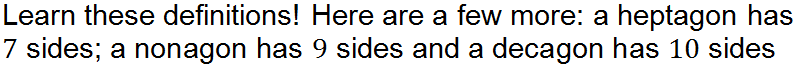Question 3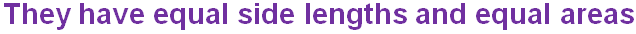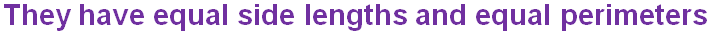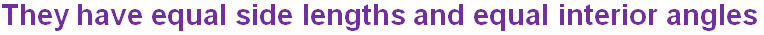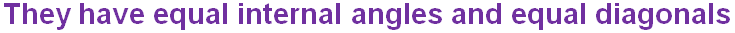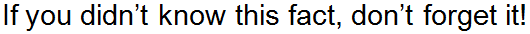Question 4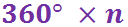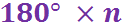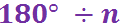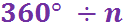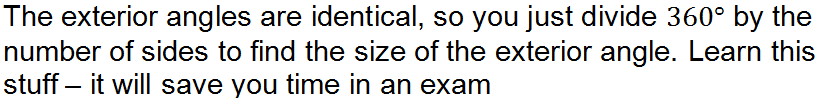Question 5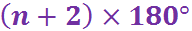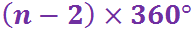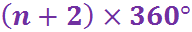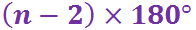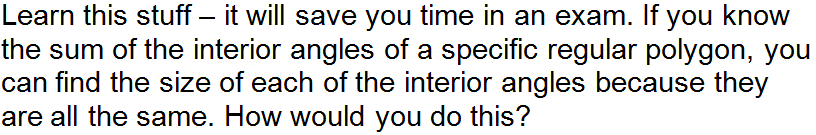Question 6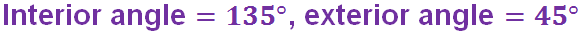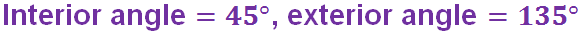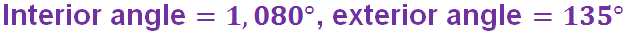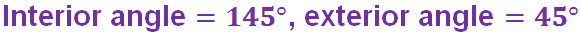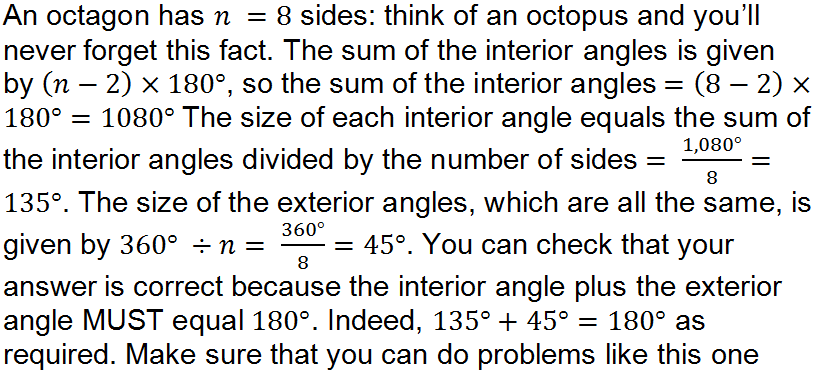Question 7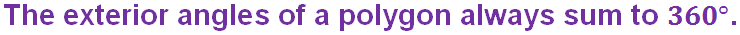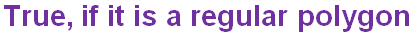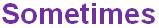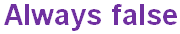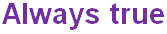Question 8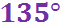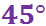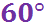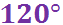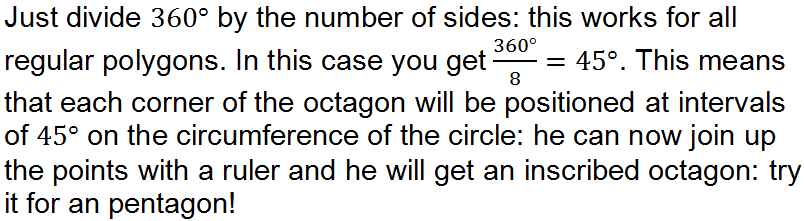Question 9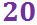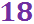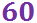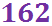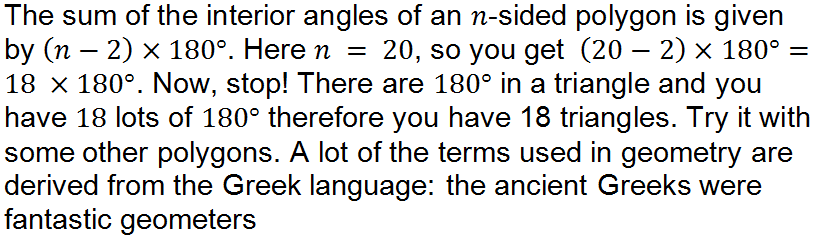Question 10